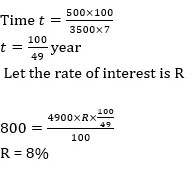# Quant Quiz for IBPS PO PRE & SBI CLERK MAINS 2019 | 6 August 2019

## Quant Quiz for IBPS PO PRE & SBI CLERK MAINS

Quant Quiz to improve your Quantitative Aptitude for SBI Po & SBI clerk exam IBPS PO Reasoning , IBPS Clerk Reasoning , IBPS RRB Reasoning, LIC AAO ,  and other competitive exams.

1. A 240 m long train takes 40 s longer to cross a platform twice its length than the time it takes to cross a pole at the same speed. What is the speed of the train?

A) 6 m/s

B) 24 m/s

C) 48 m/s

D) 12 m/s

E) None of these

Q1. Ans(D)Q2.  The distance between two stations A and B is 900 km. A train starts from A and moves towards B at an average speed of 30 km/hr. Another train starts from B, 20 minutes earlier than the train at A, and moves towards A at an average speed of 40 km/hr. How far from A will the two trains meet ?

A) 380 km

B) 320 km

C) 240 km

D) 330 km

E) None of these

Q2. Ans(A)Q3. Two trains were running in opposite directions at the speed of 48 kmph and 24 kmph respectively. If the faster train passed a man sitting in the slower train in 9.5 seconds, what is the length of the faster train?

A) 180m

B) 190m

C)  210m

D) 240m

E)  None of these

Q3. Ans(B)Q4. The length of train A is twice that of train B and speed of train A is half of that of train B. If train A crosses a man in 4 sec. then find how long will train B take to cross train A if they go in same direction.

A) 3s

B)  4s

C) 5s

D) 6s

E) None of these

Q4. Ans(D)Q5.  A basket contains 5 black and 8 yellow balls. Four balls are drawn at random and not replaced. What is the probability that they are of different colours alternatively.

A) 56/429

B) 57/429

C) 61/429

D) 68/429

E) None of these

Q5. Ans(A)Q6. Manish borrowed some money at the rate of 7 per cent per annul for the first three years, 9 per cent per annul for the next six years and 10 per cent per annul for the period beyond nine years. If the total interest paid by him at the end of fifteen years is Rs 4050, how much money did he borrow( if simple interest be reckoned)?

(a) Rs 2800

(b) Rs 3600

(c) Rs 3000

(d) Rs 3500

(e) None of these

Q6. Ans(c)Q7. The bases of a hemisphere and a cone are equal. If their heights are also equal then ratio between their curved surface area is—

A)B)C)D)E)Q7. Ans(B)Q8. On Rs 3500 invested at a simple interest rate 7 per cent per annum, Rs 500 is obtained as interest in certain years, In order to earn Rs 800 as interest on Rs 4900 in the same number of years, what should be the rate of simple interest?

(a) 9%

(b) 10%

(c) 12%

(d) 8%

(e) None of these

Q8. Ans(d)Q9. A & B working together can complete a piece of work in 72 days, B & C working together can complete the same work in 90 days and A & C working together can complete the same work in 75 days. If A, B & C starts working together, then find in how many days will the same work be completed?

A)B)C)D)E)Q1. Ans(B)10.  A takes half as long to do a piece of work as B takes, and C does it in the same time as A and B together, and if all three working together would take 7 days, how long would each take separately?

A)B)C)D)E)Q10. Ans(A)### You Can Read This Also:

Best E-books for LIC AAO 2019 : Get PDF here

ambitiousbaba.com need your support to Grow

I challenge you will get Best Content in Our PDFs with Detail solutions and Latest Pattern

Memory Based Puzzle E-book | 2016-19 Exams Covered

Get PDF here

Caselet Data Interpretation 200 Questions

Get PDF here
Puzzle & Seating Arrangement E-Book for BANK PO MAINS (Vol-1)

Get PDF here

### ARITHMETIC DATA INTERPRETATION 2019 E-book

Get PDF here
The Banking Awareness 500 MCQs E-book| Bilingual (Hindi + English)

Get PDF here

High Level DATA INTERPRETATION Practice E-BOOK

Get PDF here

### How to Access on App:-

1. Go to Playstore search Ambitious Baba or Click here to Install App